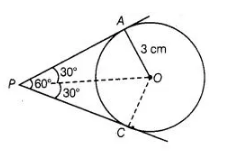# If two tangents inclined at an angle 60°

Question:

If two tangents inclined at an angle 60° are drawn to a circle of radius 3 cm, then the length of each tangent is

(a) $\frac{3}{2} \sqrt{3} \mathrm{~cm}$

(b) $6 \mathrm{~cm}$

(c) $3 \mathrm{~cm}$

(d) $3 \sqrt{3} \mathrm{~cm}$

Solution:

(d) Let P be an external point and a pair of tangents is drawn from point P and angle between these two tangents is 60°.Join $O A$ and $O P$.

Also, $O P$ is a bisector line of $\angle A P C$.

$\therefore \quad \angle A P O=\angle C P O=30^{\circ}$

Also,  $O A \perp A P$

Tangent at any point of a circle is perpendicular to the radius through the point of contact.

In right angled $\triangle O A P, \quad \tan 30^{\circ}=\frac{O A}{A P}=\frac{3}{A P}$

$\Rightarrow$ $\frac{1}{\sqrt{3}}=\frac{3}{A P}$

$\Rightarrow$$A P=3 \sqrt{3} \mathrm{~cm}$

Hence, the length of each tangent is $3 \sqrt{3} \mathrm{~cm}$.